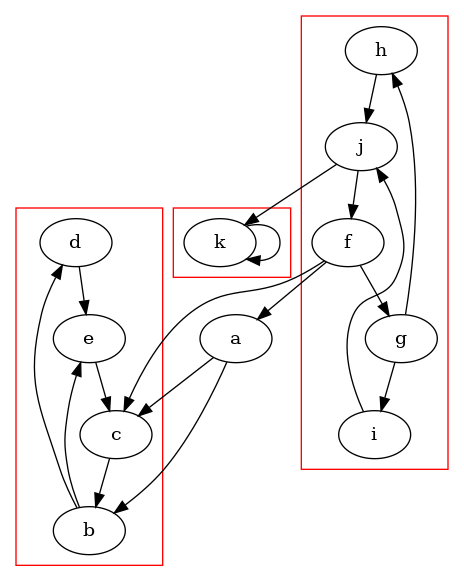Nuanced Pumpkin Mavens

# npm

## tarjan-graph3.0.0 • Public • Published

# tarjan-graph

This is a simple directed graph lib, mostly just for checking if a directed graph contains a cycle. It uses Tarjan's algorithm for checking if the graph contains a cycle.

This library also has some very basic Graphviz support for visualizing graphs using the DOT language.

## Installation

Install using `npm`:

``````npm install tarjan-graph
``````
• Note: as of v1.0.0 this library requires node >= v8.0.0. Use v0.3.0 for node < v8.0.0.
• Note: as of v3.0.0 this library was ported to TypeScript and the default export changed to get with the times. So now instead of this:
```const Graph = require('tarjan-graph');  // js
import Graph = require('tarjan-graph'); // ts```
you now do this:
```const Graph = require('tarjan-graph').default; // js
import Graph from 'tarjan-graph';              // ts```

## Usage

JavaScript:

`const Graph = require('tarjan-graph').default;`

TypeScript:

`import Graph from 'tarjan-graph';`

All examples use the following graph:

```node -e "
const Graph = require('tarjan-graph').default;

const graph = new Graph()
.add('f', ['c', 'a', 'g'])

console.log(graph.toDot());
" | dot -o docs/example-graph.png -Tpng````Doing stuff with cycles:

```console.log(graph.hasCycle());
//true
console.log(graph.getCycles());
// [
//   [ { name: 'b', successors: [...] }, { name: 'e', ... }, ... ],
//   ...
// ]

//an edge would create a cycle```

Doing stuff with SCCs:

```//same as graph.getCycles() except includes single vertices
console.log(graph.getStronglyConnectedComponents());```

Searching:

```//depth-first search (pre-order)
graph.dfs('g', (v) => {
console.log(v.name + ': ' + v.successors.map(w => w.name).join(', '));
});
/*
g: i, h
h: j
j: k, f
f: g, a, c
c: b
b: e, d
d: e
e: c
a: c, b
k: k
i: j
*/

//retrieve descendants
console.log(graph.getDescendants('a'));
//[ 'b', 'd', 'e', 'c' ]```

And of course, dat dot:

```console.log(graph.toDot());
/*
digraph {
subgraph cluster0 {
color=red;
d; c; e; b;
}
subgraph cluster1 {
color=red;
k;
}
subgraph cluster2 {
color=red;
h; f; j; i; g;
}
b -> e
b -> d
c -> b
a -> c
a -> b
d -> e
e -> c
g -> i
g -> h
f -> g
f -> a
f -> c
h -> j
i -> j
j -> k
j -> f
k -> k
}
*/```

## Keywords

none

### Install

`npm i tarjan-graph`

### Repository

github.com/tmont/tarjan-graph

6,546

3.0.0